Home

# A revisit of the equilibrium assumption for predicting near-wall turbulence

## Abstract

In this study, we revisit the consequence of assuming equilibrium between the rates of production (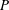$P$ ) and dissipation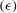$({\it\epsilon})$ of the turbulent kinetic energy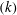$(k)$ in the highly anisotropic and inhomogeneous near-wall region. Analytical and dimensional arguments are made to determine the relevant scales inherent in the turbulent viscosity (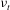${\it\nu}_{t}$ ) formulation of the standard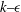$k{-}{\it\epsilon}$ model, which is one of the most widely used turbulence closure schemes. This turbulent viscosity formulation is developed by assuming equilibrium and use of the turbulent kinetic energy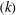$(k)$ to infer the relevant velocity scale. We show that such turbulent viscosity formulations are not suitable for modelling near-wall turbulence. Furthermore, we use the turbulent viscosity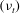$({\it\nu}_{t})$ formulation suggested by Durbin (Theor. Comput. Fluid Dyn., vol. 3, 1991, pp. 1–13) to highlight the appropriate scales that correctly capture the characteristic scales and behaviour of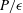$P/{\it\epsilon}$ in the near-wall region. We also show that the anisotropic Reynolds stress (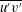$\overline{u^{\prime }v^{\prime }}$ ) is correlated with the wall-normal, isotropic Reynolds stress (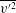$\overline{v^{\prime 2}}$ ) as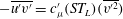$-\overline{u^{\prime }v^{\prime }}=c_{{\it\mu}}^{\prime }(ST_{L})(\overline{v^{\prime 2}})$ , where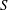$S$ is the mean shear rate,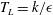$T_{L}=k/{\it\epsilon}$ is the turbulence (decay) time scale and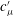$c_{{\it\mu}}^{\prime }$ is a universal constant. ‘A priori’ tests are performed to assess the validity of the propositions using the direct numerical simulation (DNS) data of unstratified channel flow of Hoyas & Jiménez (Phys. Fluids, vol. 18, 2006, 011702). The comparisons with the data are excellent and confirm our findings.

## References

Hide All
MathJax
MathJax is a JavaScript display engine for mathematics. For more information see http://www.mathjax.org.

# A revisit of the equilibrium assumption for predicting near-wall turbulence

## Metrics

### Full text viewsFull text views reflects the number of PDF downloads, PDFs sent to Google Drive, Dropbox and Kindle and HTML full text views.

Total number of HTML views: 0
Total number of PDF views: 0 *Loading metrics...

### Abstract viewsAbstract views reflect the number of visits to the article landing page.

Total abstract views: 0 *Loading metrics...

* Views captured on Cambridge Core between <date>. This data will be updated every 24 hours.

Usage data cannot currently be displayed.